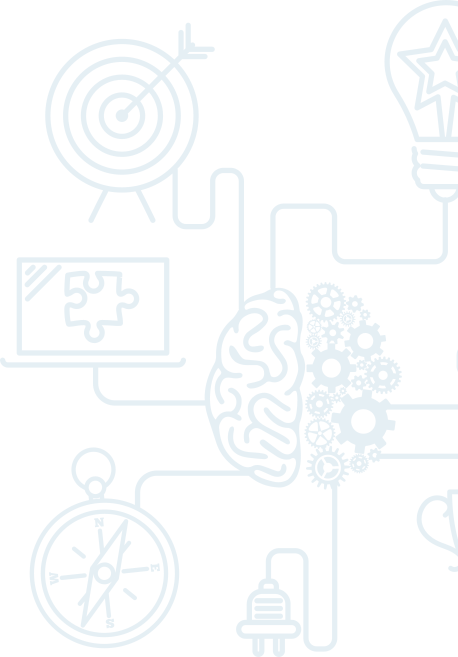# Foundations - Math & Problem Solving

After many inquiries and requests for a Math & Problem Solving program for students in Grades 3 – 4, we have developed a program for this age group that will prepare students for our Level One program.

The Foundations in Math & Problem Solving program addresses the following topics:

• All four arithmetical operations on whole numbers (2, 3, 4 digits). Note: we will ensure  our students know their multiplication tables well.
• All four operations on decimals (two digits after decimal point)
• Problem solving using four arithmetical operations; one and two step processes
• Development of logical thinking / reasoning through various problem solving opportunities and many dynamic brain teasers
• Elements of Geometry
• Games of Logic